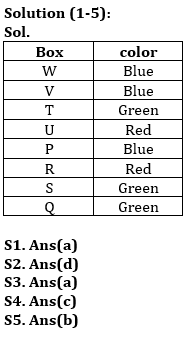Latest Banking jobs   »

# Reasoning Quiz For ECGC PO 2023 -18th May

Directions (1-5): Study the information carefully and answer the questions given below.
Eight boxes are placed in a stack one above another. All boxes are of three colors i.e., Red, Blue, Green (but not necessary in same order). Not more than three boxes are same color.
Two boxes are placed between U and S. Three boxes are placed between U and Q, which is green color box. Only Box R and the box which is placed just above box P are red color. Box V is not green color. One box is placed between box U and box V, which is not placed just above or just below box S. One box is placed between box Q and red color Box. Box Q is not placed just above box S. Box R is placed below box P. Box W is blue color box which is placed above box U but not just above. Box P is blue color. Box S is not blue color. Only one box is placed between W and T, which is not blue color box. Box Q is placed below box U.

Q1. Which of the following color is of box U?
(a) Red
(b) Blue
(c) Green
(d) Either Red or green
(e) Either Red or Blue

Q2. How many boxes are placed between Box Q and T?
(a) Two
(b) Three
(c) One
(d) Four
(e) None

Q3. Which of the following box is placed just above box R?
(a) P
(b) T
(c) V
(d) S
(e) None of these

Q4. Which of the following combination is true?
(a) V-Red
(b) T-Red
(c) U-Red
(d) S-Red
(e) None is true

Q5. Which of the following pair of boxes is of green color?
(a) T, Q, U
(b) Q, T, S
(c) S, T, V
(d) T, U, S
(e) None of these

Directions (6-10): These questions are based on the following six numbers.

843 158 425 674 362 568

Q6. If we add 2 to the second digit of each number and subtract 1 from the first digit of each number, then which of the given will become the lowest number?
(a) 362
(b) 568
(c) 158
(d) 425
(e) 843

Q7. If all the digits in each of the numbers are written in increasing order within the number, then which of the given number will become the second highest?
(a) 674
(b) 568
(c) 425
(d) 362
(e) 158

Q8. If the first two digits of each number are interchanged, then which of the following number will become the second lowest number?
(a) 674
(b) 158
(c) 568
(d) 843
(e) 362

Q9. In each number, if we subtracted 1 from second digit and then interchange with last digit, then which among these number will be 2nd lowest number?
(a) 425
(b) 158
(c) 674
(d) 362
(e) 843

Q10.If we replace all the even digits in each number with zero, then which of the following number will become the second lowest?
(a) 674
(b) 425
(c) 843
(d) 158
(e) 568

SolutionsSolutions (6-10):
S6. Ans.(c)
S7. Ans.(a)
S8. Ans.(d)
S9. Ans.(d)
S10. Ans.(b)## FAQs

### What is the total duration of ECGC PO for objective?

The duration of ECGC PO for objective is 140 minutes

#### Congratulations!Union Budget 2023-24: Free PDF